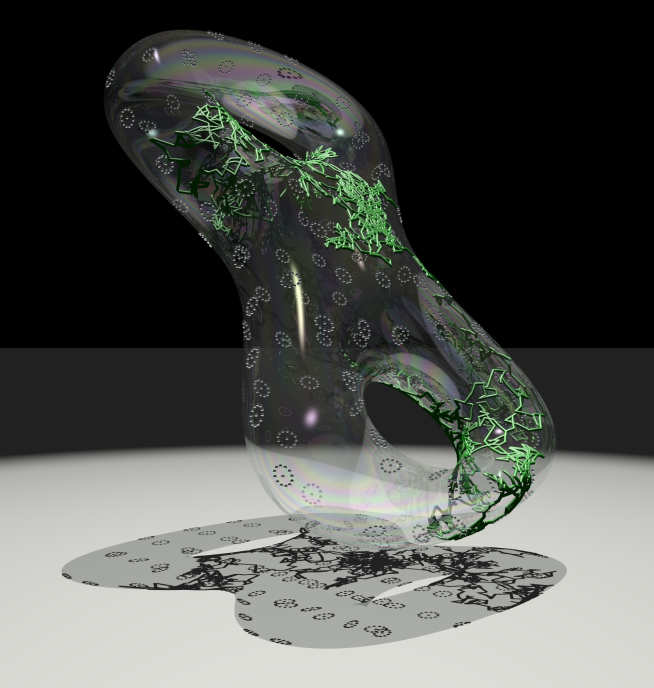# OverviewBrownian motion on a genus 2 surface

The theme of the workshop is the use of geometric methods in Stochastic Analysis and Statistics, with a particular focus on the following topics.

Geometry and Stochastic Analysis: The UK is at the forefront of rough paths theory from the founding work of Terry Lyons at Oxford through to the recent Fields medal winning work of Martin Hairer which applied techniques from rough paths to give rigorous foundations to important stochastic partial differential equations of mathematical physics (the KPZ and 3-dimensional stochastic quantization equations). To understand these equations, important new theories have been developed: those of regularity structures and of paracontrolled distributions, the theory of controlled rough paths providing an important ingredient in both. In addition to the ones in mathematical physics, rough path theory has found applications in statistics, machine learning and finance. The workshop will bring together researchers interested both in the pure mathematical developments and in their applications.

Information Geometry: Mark Girolami and his co-authors proposed a Riemannian Manifold Hamiltonian Monte Carlo sampler to resolve the shortcomings of existing Monte Carlo algorithms when sampling from target densities that may be high dimensional and exhibit strong correlations. This approach exploits the Riemannian geometry of statistical manifolds to optimise scaling behaviour and results allow efficient sampling even in high dimensions. The study of Hamiltonian Monte Carlo is growing rapidly and has interactions with the study of stochastic analysis on manifolds. Stochastic processes on statistical manifolds have also been discussed by Brigo et al. in connection with dimensionality reduction of stochastic filtering schemes based on projections. The notion of projection for stochastic differential equations poses questions that are not appreciated in the classical setting of ordinary differential equations. Adopting the perspective of Rough Differential Equations to tackle such questions is an emerging area of research, currently being explored through collaborations among UK researchers such as D. Brigo, M. Girolami and G. Pavliotis, N. Newton and T. Cass.

Variational Approach to Fokker-Planck: Since the seminal paper by Jordan, Kinderlehrer and Otto, the Fokker-Planck equation dynamics has been regarded as a gradient flux, or a steepest descent, for free energy with respect to the Wasserstein metric. This has opened a new area in PDEs research and many results related also to optimal transport have been formulated. Potential connections with the two areas above are becoming clear among researchers, especially with optimal approximation of the Fokker-Planck equation and  with Hamiltonian Monte Carlo, leveraging on the common geometry techniques and metrics.